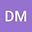A Comparison of Quantum and Traditional Fourier Transform Computations
•• Damian Musk
Damian Musk
Author Profile## Abstract

The quantum Fourier transform (QFT) can calculate the Fourier transform of a vector of size $$N$$ with time complexity $$\mathcal{O}(\log^{2}N)$$ as compared to the classical complexity of $$\mathcal{O}(N\log N)$$. However, if one wanted to measure the full output state, then the QFT complexity becomes $$\mathcal{O}(N\log^{2}N)$$, thus losing its apparent advantage, indicating that the advantage is fully exploited for algorithms when only a limited number of samples is required from the output vector, as is the case in many quantum algorithms. Moreover, the computational complexity worsens if one considers the complexity of constructing the initial state. In this paper, this issue is better illustrated by providing a concrete implementation of these algorithms and discussing their complexities as well as the complexity of the simulation of the QFT in MATLAB.

14 Apr 2020Submitted to Computing in Science and Engineering
14 Apr 2020Editor invited a reviewer
14 Apr 2020Editor invited a reviewer
11 May 2020Reviewer #1 has been removed by the editor
17 May 2020Review Report #1 received
28 Jul 2020Reviewer #2 has been removed by the editor
21 Nov 2020Published in Computing in Science and Engineering
01 Nov 2020Published in Computing in Science & Engineering volume 22 issue 6 on pages 103-110. 10.1109/MCSE.2020.3023979
10.22541/au.159795474.47457948
Matthias Troyer posted a review
The manuscript "A Comparison of Quantum and Traditional Fourier Transform Computations" discusses a very important and often overlooked aspect of quantum computing, namely a fair and detailed comparison of a quantum algorithm, its classical simulation, and its classical counterpart taking into account the complexity of I/O. Such an article is valuable and worth publishing. The current manuscript, however, still contains some inaccuracies that should be fixed and I will make suggestion on how the presentation can be improved.Let me first summarize the main result in